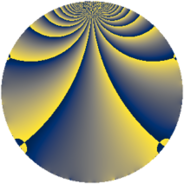# Properties

 Label 1560.1.dkLevel $1560$ Weight $1$ Character orbit 1560.dk Rep. character $\chi_{1560}(1109,\cdot)$ Character field $\Q(\zeta_{6})$ Dimension $8$ Newform subspaces $2$ Sturm bound $336$ Trace bound $3$

# Related objects

## Defining parameters

 Level: $$N$$ $$=$$ $$1560 = 2^{3} \cdot 3 \cdot 5 \cdot 13$$ Weight: $$k$$ $$=$$ $$1$$ Character orbit: $$[\chi]$$ $$=$$ 1560.dk (of order $$6$$ and degree $$2$$) Character conductor: $$\operatorname{cond}(\chi)$$ $$=$$ $$1560$$ Character field: $$\Q(\zeta_{6})$$ Newform subspaces: $$2$$ Sturm bound: $$336$$ Trace bound: $$3$$

## Dimensions

The following table gives the dimensions of various subspaces of $$M_{1}(1560, [\chi])$$.

Total New Old
Modular forms 24 24 0
Cusp forms 8 8 0
Eisenstein series 16 16 0

The following table gives the dimensions of subspaces with specified projective image type.

$$D_n$$ $$A_4$$ $$S_4$$ $$A_5$$
Dimension 8 0 0 0

## Trace form

 $$8 q + 4 q^{4} - 4 q^{9} + O(q^{10})$$ $$8 q + 4 q^{4} - 4 q^{9} - 4 q^{16} - 8 q^{25} - 4 q^{30} + 4 q^{36} + 4 q^{39} + 12 q^{46} + 4 q^{49} - 4 q^{55} - 8 q^{64} - 8 q^{66} - 8 q^{79} - 4 q^{81} - 4 q^{94} + O(q^{100})$$

## Decomposition of $$S_{1}^{\mathrm{new}}(1560, [\chi])$$ into newform subspaces

Label Dim $A$ Field Image CM RM Traces $q$-expansion
$a_{2}$ $a_{3}$ $a_{5}$ $a_{7}$
1560.1.dk.a $4$ $0.779$ $$\Q(\zeta_{12})$$ $D_{6}$ $$\Q(\sqrt{-30})$$ None $$0$$ $$-2$$ $$0$$ $$0$$ $$q+\zeta_{12}q^{2}+\zeta_{12}^{4}q^{3}+\zeta_{12}^{2}q^{4}+\zeta_{12}^{3}q^{5}+\cdots$$
1560.1.dk.b $4$ $0.779$ $$\Q(\zeta_{12})$$ $D_{6}$ $$\Q(\sqrt{-30})$$ None $$0$$ $$2$$ $$0$$ $$0$$ $$q-\zeta_{12}q^{2}-\zeta_{12}^{4}q^{3}+\zeta_{12}^{2}q^{4}+\zeta_{12}^{3}q^{5}+\cdots$$